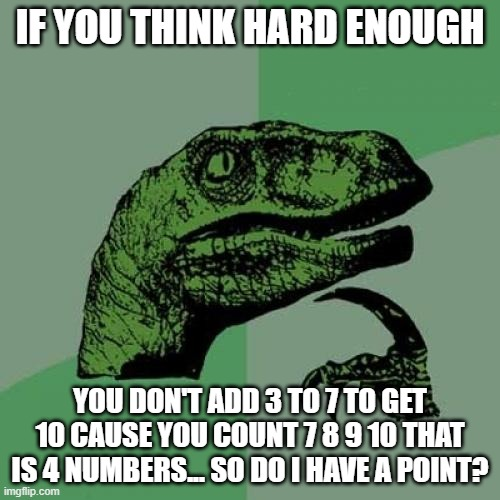# Does Math, counting, and adding lie to us??shareCaption this Meme
Post Comment
Best first
3 ups, 7m,
No. Ten is the result. you count three to reach ten.
I can tell you another secret, the sum of 6 to 10 is 40.
And any number that is divisible by three can be tested by adding all the digits:
123 = 1 + 2 + 3 = 6, so 123 is divisible by three without a remainder.
124 = 1 + 2 + 4 = 7, therefore 124 is not divisable by three without a remainder.Caption this Meme